## How to work out the dynamic range of a filter empiracallyStarted by 7 years ago16 replieslatest reply 7 years ago987 views

I have a filter that I am unable to analyse analytically to derive its dynamic range so I would like to analyse it by observing it. The filter performs a decimation by N (with appropriate low pass filtering), takes signed 16 bit integer inputs and has 32 bit signed integer outputs.

I can insert any frequency of any magnitude into the filter. Any advice on a procedure to measure the DR would be appreciated.

[ - ]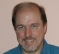I assume by "dynamic range" you mean "maximum gain".  I would use max(abs(fft(impulseResponse,veryLargeFFTSize)))

Sampling-rate converters are linear, time-varying filters.  If the aliasing is insignificant (as it should be in any good decimator), then there should be no surprises.

[ - ]This is defiantly a linear with insignificant aliasing. I was thinking that dynamic range was: "the ratio of the largest to the smallest intensity of sound that can be reliably transmitted or reproduced by a particular sound system, measured in decibels."

So I guess my question should really be how do I detect the smallest signal I can get through my filter?

[ - ]Everyone uses the term "dynamic range" differently, so it's good to either mention what service you're talking about, or go ahead and define it.

If you're just interested in determining the smallest signal you can detect, run signals through it and see how much they're corrupted.  I would expect that you'll be able to detect a signal at a much lower level than you'd find it pleasant -- you may be more interested in defining your dynamic range as the ratio of the biggest signal to the worst-case quantization noise.

[ - ]Hi andrewstanfordjason. Your question has very deep and subtle implications. That's because multirate (decimation) filters do not have a frequency response in the normal sense of what we call the frequency response of a single-rate filter. I say that because multirate filters do not have a "one-to-one" correspondence between a single input frequency and that frequency at the output of the filter. (For that reason multirate filters also do not have z-domain transfer functions.)

Add to this unpleasant situation the fact that multirate filters are time-variant so traditional Fourier analysis is not valid for analyzing the frequency-domain behavior of such filters.

I've tried estimating the frequency response of decimation filters using both wideband chirp and wideband noise input signals. (By wideband I mean from zero Hz to Fs/2 Hz where Fs is the input sample rate.) But those frequency response estimations suffer from aliasing. If you want to measure the lowest-amplitude input signal that you can detect at the decimation filter's output, I suggest you apply a medium-amplitude sine wave, whose freq is between zero Hz and half the output sample rate, to your filter and detect (recognize) that sine wave at the filter's output. Then slowly decrease the input sine wave's amplitude until you can no longer detect that sine wave at the output of your filter.

Good Luck.
[ - ]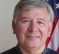If one were to define the dynamic range as "from zero to the maximum input level without x% distortion as a function of frequency" then you could apply sinusoids at various frequencies at at various levels, FFT the output and measure the 2nd and 3rd harmonic components (and 4th and 5th if you like) and determine the amount of harmonic distortion.  Or, perhaps better would be to compare the frequency amplitude at the fundamental vs. the total output.  That takes care of the upper end vs. freq.

Then, as others have suggested, you might apply low level signals to determine the minimum signal necessary to achieve some SNR at the output.  Or perhaps you might apply a similar approach using an FFT as above.

If you then define the dynamic range as the difference between the maximum acceptable input signal and the minimum, you will likely find that the maximum signal level dominates the value for dynamic range.  At least that's my guess.

I can imagine a plot at a single frequency of the output of the filter as a function of single-frequency sinusoid input.  For very low levels of input you won't be able to tell the amplitude or the amplitude will be pure noise of a single amplitude.  So the curve will be flat.  For very high levels of input, the output amplitude will be flat also; due to saturation.  The result is a rather S-shaped curve with flat ends.  The difference between the flat portions *could be* defined as "dynamic range" or so many dB below the flats could be so defined.

Then you make this determination over the frequency range to f>0 to f<=Fs/2.

Is that the sort of thing you meant to do?

[ - ]Tell us about the filter and maybe someone (maybe even someone named Tim) will help you to figure out how to analyze it analytically.

[ - ]The filter is a polyphase symmetric FIR with varying coefficient quantisation, i.e. each coefficient has 14~16 bits of information(mantissa?). In effect the coefficients are like floating point numbers but there is an exponent common for a block of say N coefficients, i.e. the first N coefficients share an exponent the the next N share one and so on. Hope that helps.

[ - ]Wow.  That would certainly make things tedious, and may mean it's better to do it by experiment after all.  Do you know every possible set of coefficients, or are they computed on line?

What dgshaw6 suggests for figuring out the maximum signal.

For the quantization noise, you can probably figure that it'll have an RMS value of $${\frac{N}{12}}\mathrm{LSB}$$, where $$N$$ is the number of taps in the filter and $$\mathrm{LSB}$$ is the weight of the least significant bit after multiplication.

The coefficient quantization won't cause additive noise, but it might cause oddball multiplicative noise that I'm not sure how to predict.  I'd probably try to at least find an upper bound on the noise, but unless you want to pick out a weak signal in the presence of a strong one, I suspect it won't cause much problem.

[ - ]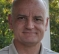This will tell you the largest result that you could ever get at the output, because it assumes that the samples are at saturation, and happen to have same sign as the individual coefficients.

Obviously, this will give you a value greater than 32 bits, but it will also tell you what sort of rms level you can use for the data inputs without overflowing 32 bits on average.

Then you can do the same thing with sum(abs(coefficients)*1) and see if this value even reaches the threshold of the 32 bit number that you plan to preserve.

Some off the wall thoughts.

David

[ - ]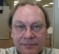I agree with Tim: define what "you" mean by dynamic range. Often defining a problem exposes the solution.

Generically, dynamic range is taken to mean the peak-to-peak-ness (P2P) of something, but this is way different from "useful range of inputs" which includes the impact of small signal. P2P is important to prevent digital filter clipping (and saturation for analog inputs). Of course, start with the levels of the input (analog first if digitized by some A/D process).

The useful range of inputs includes considerations for SNR which falls quickly for small signals. SNR has a strong influence on the accuracy of the signal's magnitude (i.e. if 4 bits P2P for 16 bit dynamic range processing, then very likely quantization will dominate the error vs. "true" signal noise. This may require cascading sections with adjustable gain (e.g. AGC) to keep the signal level high enough to reduce quantization. If it's over-sampled, then decimation filtering could improve SNR if all noise sources are zero-mean Gaussian.

Some of this is related to signal and architecture. For an AM signal, add a "DC block" after detection (whether analog or digital) to remove the DC (carrier level) and add AC gain to increase the AC part's dynamic range and hence improve SNR.

Once a signal is corrupted by clipping/saturation or quantization noise, it is lost forever.

[ - ]Agreed, I will do some reading so that I can better describe what I am trying to define.

My feeling is that I would like to be able to know that a signal from 0 dBFS to -X dBFS can be inputted and the filtered result can be observed on the output. Any input signal smaller the -X dB will be just noise.

However, I also feel that that statement might be totally wrong!

Thanks

[ - ]Hi Andrew,

On the one hand, I believe that the dynamic range is dictated by the input signal mainly, since the filter itself "ideally speaking" has a gain of 1. The sum of the FIR filter coefficients shall be around 1. On the other hand, the fixed-point filter coefficients dynamic range is dictated by the bit-width of the coefficients themselves. As an example, if you have a filter coefficient with 4-bits, then the smallest step (or smallest detectable signal) is [1/2^4 = 0.0625], while if you have a filter coefficient with 16-bit resolution then the smallest step is [1/2^16 = 0.00000152587]. So, I totally agrees with Tim's reply.

Good luck.

Regards.

Ahmed.

[ - ]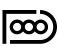You can measure DR using a sinewave of fixed frequency and varying amplitude from MAX to 0. You use a notch filter (or FFT) to analyze the processed output.

The point of time when the output gives less than 1% THD+N is the "full-scale" (from AES-17 definition), the point of time when you cannot any more detect your sinewave gives the DR with respect to full-scale.

[ - ]Hi Andrew,

I just forgot to mention something in my previous reply. Matlab has an option within the FDATOOL called "Maximize Dynamic Range" which is available at the coefficients export tab. However, when I checked the outcome of it, I am not sure if I would recommend it.

This process is simply normalizing the filter coefficients (COEFF./max[COEFF]). If you test these set of coefficients it is simply adding a gain to the filter. Therefore, I am not sure if this is what you are looking for, or what you meant.

Regards.

Ahmed.

[ - ]To further explain what I am trying to achieve: I am implementing a polyphase decimation FIR, say divide by 8, with a 16x16 multiply accumulate to a 32 bit accumulator all with integer arithmetic. I require greater than 104dB of dynamic range. If all my coefficients were to be of the same q format then, I assume, the dynamic range would be limited to 6.02 * 16 = 96ish dB. To solve this I plan on implementing(something to the effect of):

//first half of FIR

first_sum = second_sum = 0;

for (unsigned i=0;i<N/2;i++){

first_sum += (coef[i]*data[i]);

first_sum >>= 1

}

//second half of FIR

for (unsigned i=0;i<N/2;i++){

second_sum += (coef[N - 1 - i]*data[N - 1 - i]);

second_sum >>= 1

}

return first_sum + second_sum;

Obviously, if I were to implement this for real there would be lots of implementation details to deal with but the idea is:

• The outer most(smallest) coefficients are pre-scaled by more then the centre coefficients,
• as the filter progresses along the data occasionally the q format of the previous coefficient will be too small to hold the current one and the format will have to change(along with the accumulator),
• as this is symmetric, with the greatest values in the centre, it has to happened from the ends in .

I have seen good results with this so far but I am looking to prove it works.

Also, thanks everyone for your input, much appreciated.

[ - ]